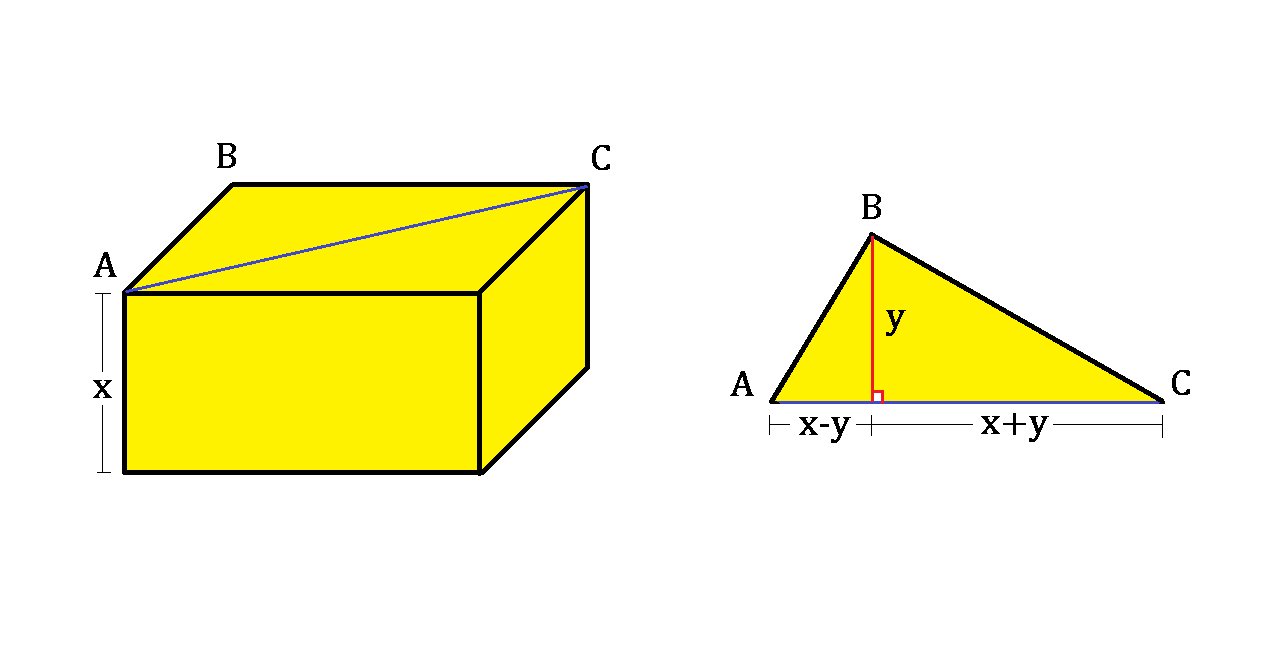# Factorize the BoxA cuboid box has a height of $x$ and a base diagonal (in blue) of length $2x$.

Considering the triangle $ABC$ separately, its height (in red) is $y$, partitioning the (blue) base into $x-y$ and $x+y$, as shown above right.

If the volume of the box is 108, what is the value of $y$?


Hint: This wiki can help.

×